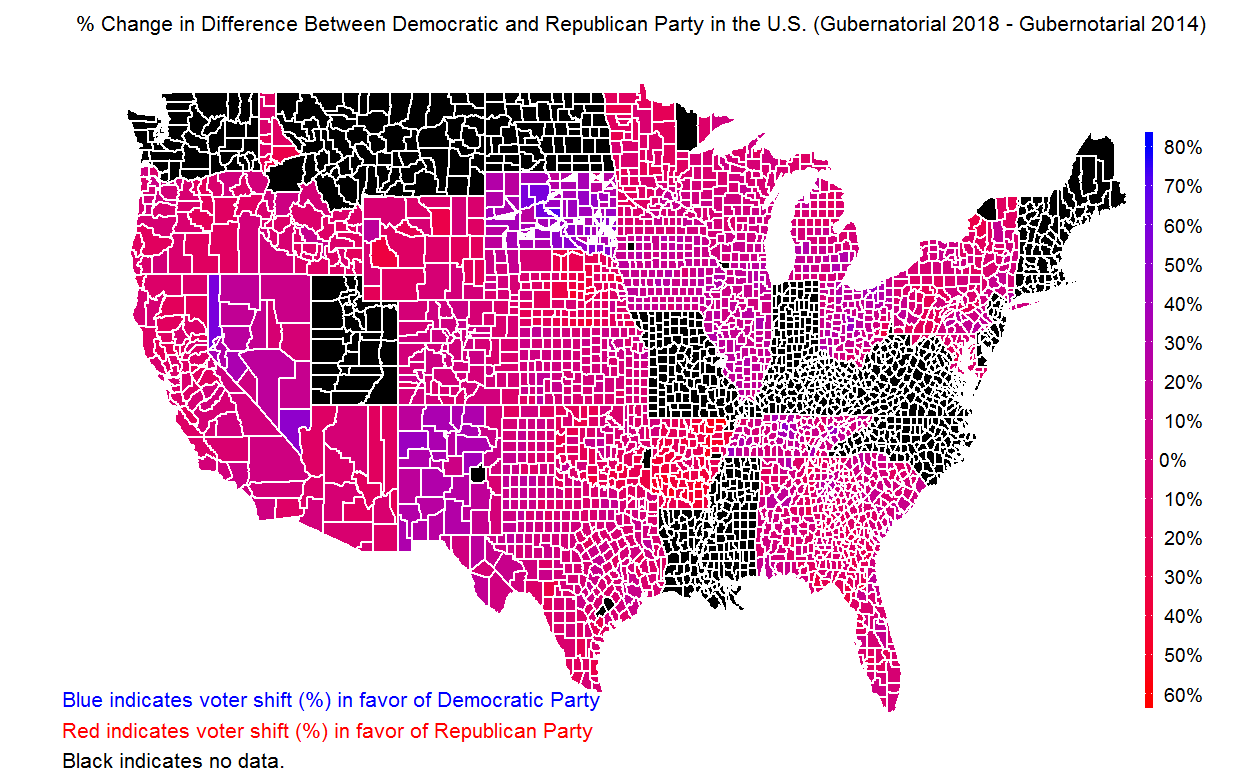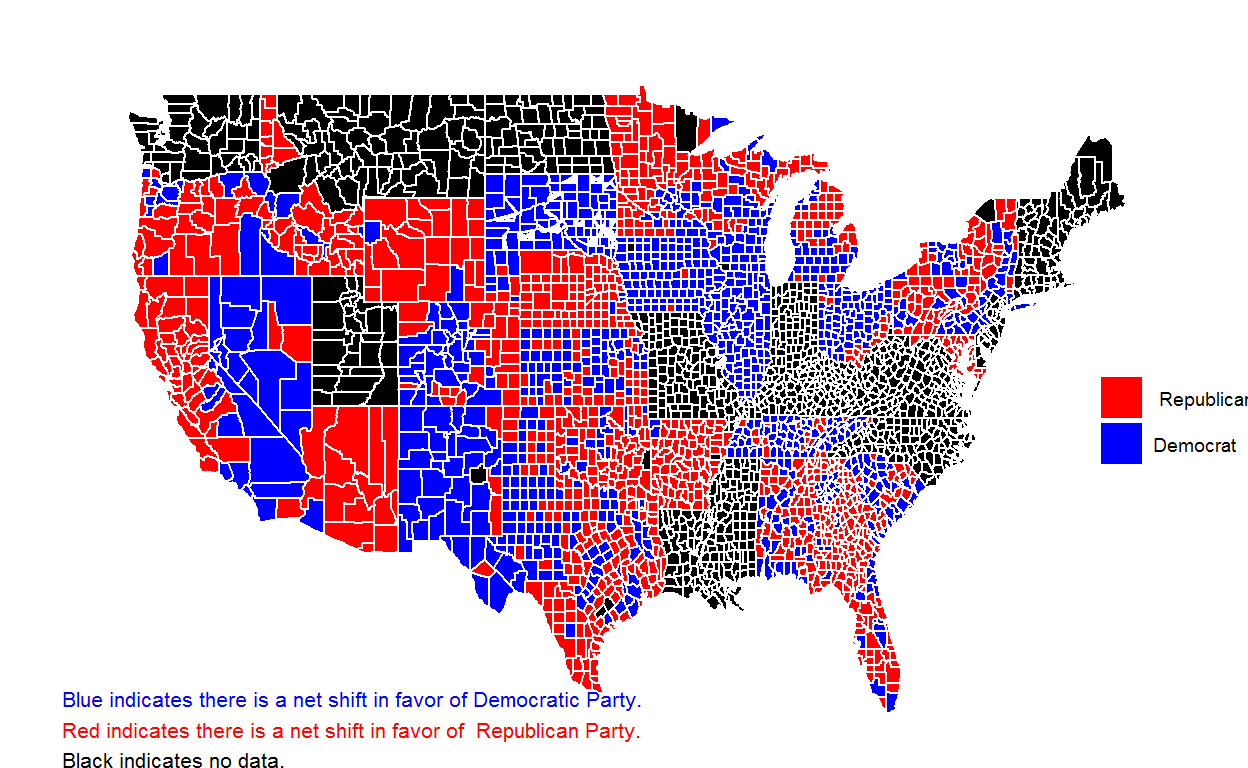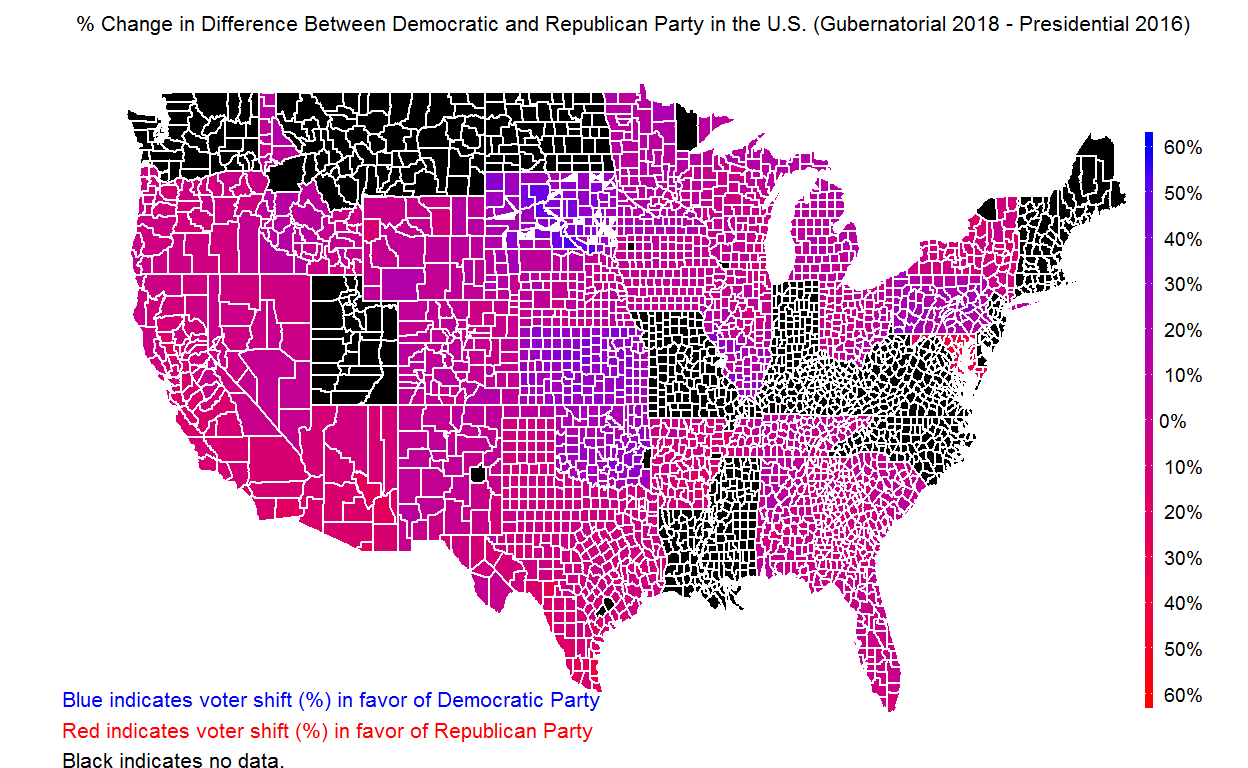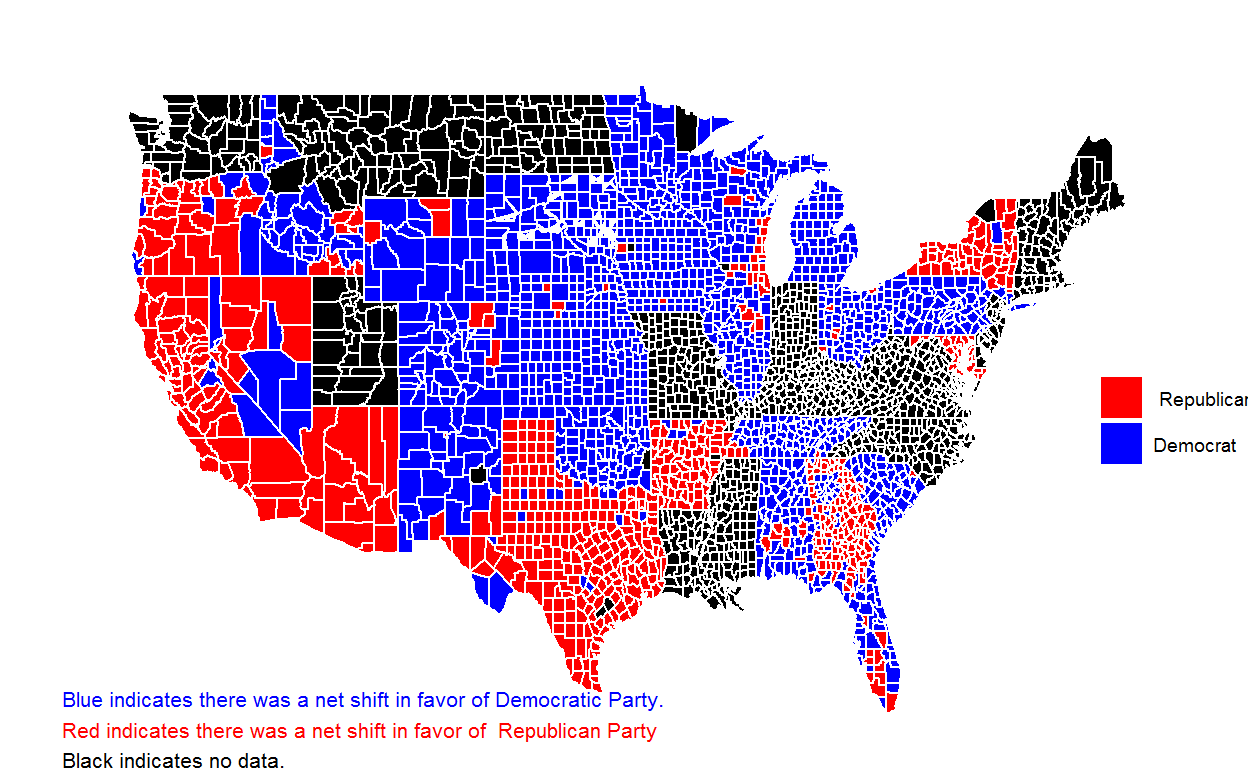# Mapping the Election 2018 Data

###### A Quick Look at the Election 2018 Data
November 12, 2018
###### How Does Extreme Gradient Boosting (XGBoost) Work?
January 15, 2019
Mapping the Election 2018 Data

# Mapping the Election 2018 Data

In this post, I tried to create county level maps to understand the change in difference between two parties from Gubernatorial 2014 election to Gubernatorial 2018 election or from Presidential 2016 election to Gubernatorial 2018 election. In order to reproduce the plots and numbers on this post, you have to download the following two datasets. I provide the R code for reproducing the numbers and plots at the end of each section.

I calculated a variable to compare 2014 and 2018 gubernatorial elections and to compare 2016 presidential and 2018 gubernatorial elections at the county level. This variable is the change in % difference between democratic and republican party (dem - rep). Positive numbers indicates there was a net shift in favor of Democratic party and negative numbers indicate there was a net shift in favor of Republican party.

For instance, suppose Democrats got 55% and Republicans got 45% of the vote in the 2014 Gubernatorial elections. So, there was a 10% difference in favor of Democrats. In 2018, Democrats got 40% and Republicans got 60% of the vote. So, there was a 20% difference in favor of Republicans. This means there is a 30% shift in favor of Republicans.

In another example, for instance, suppose Democrats got 60% and Republicans got 40% of the vote in the 2016 Presidential election. So, there was a 20% difference in favor of Democrats. In 2018 gubernatorial election, Democrats got 65% and Republicans got 35% of the vote. So, there was a 30% difference in favor of Democrats. This means there is a 10% shift in favor of Democrats.

In addition to computing this difference, I also created a binary variable based on this variable. (0) indicates there is a net shift in favor of Republicans and (1) indicates there is a net shift in favor of Democrats.

Below are the county level plots for these variables and the R code to produce these plots.

## Gubernatorial 2018 - Gubernatorial 2014 (US)library(ggplot2)
library(maps)
library(mapdata)
library(Hmisc)
library(grid)
options(scipen=999)
##############################
past    <- read.csv("https://raw.githubusercontent.com/MEDSL/2018-elections/master/election-context-2018.csv")
present <- read.csv("election2018.csv")
d       <- merge(past,present,by=c("state","county"),all=TRUE)
####################################

d$total.vote2016pres <- d$trump16 + d$clinton16 + d$otherpres16
d$total.vote2014gov <- d$demgov14 + d$repgov14 + d$othergov14
d$total.vote2018gov <- d$demgov18 + d$repgov18 + d$othergov18
d$total.vote2018sen <- d$repsen18 + d$demsen18 + d$othersen18

d$p.trump16 <- d$trump16/d$total.vote2016pres d$p.clinton16 <- d$clinton16/d$total.vote2016pres
d$p.demgov14 <- d$demgov14/d$total.vote2014gov d$p.repgov14  <- d$repgov14/d$total.vote2014gov
d$p.demgov18 <- d$demgov18/d$total.vote2018gov d$p.repgov18  <- d$repgov18/d$total.vote2018gov

d$p.diffgov14 <- d$p.demgov14 - d$p.repgov14 d$p.diffgov18  <- d$p.demgov18 - d$p.repgov18
d$p.diffpres16 <- d$p.clinton16- d$p.trump16 d$p.diffgov     <- (d$p.diffgov18 - d$p.diffgov14 )*100
d$p.diffpresgov <- (d$p.diffgov18 - d$p.diffpres16 )*100 d$county <- as.character(d$county) d$state <- as.character(d$state) d <- d[,c("state","county","p.diffgov","p.diffpresgov")] all.counties <- map_data("county") all.counties$region    <- capitalize(all.counties$region) all.counties$subregion <- capitalize(all.counties$subregion) colnames(all.counties)[5:6] <- c("state","county") # Below are just some fixes to standardize the state and county names between two datasets d = d[-which(d$state=="Hawaii"),]
d = d[-which(d$state=="Alaska"),] d[which(d$state=="District of Columbia"),]$county = "Washington" all.counties[which(all.counties$state=="District of columbia"),]$state = "District of Columbia" all.counties[which(all.counties$state=="New hampshire"),]$state = "New Hampshire" all.counties[which(all.counties$state=="New jersey"),]$state = "New Jersey" all.counties[which(all.counties$state=="New mexico"),]$state = "New Mexico" all.counties[which(all.counties$state=="New york"),]$state = "New York" all.counties[which(all.counties$state=="North carolina"),]$state = "North Carolina" all.counties[which(all.counties$state=="North dakota"),]$state = "North Dakota" all.counties[which(all.counties$state=="Rhode island"),]$state = "Rhode Island" all.counties[which(all.counties$state=="South carolina"),]$state = "South Carolina" all.counties[which(all.counties$state=="South dakota"),]$state = "South Dakota" all.counties[which(all.counties$state=="West virginia"),]$state = "West Virginia" states = unique(all.counties$state)

# For every state, check the consistency of county names
# fix any inconsistency

for(i in 1:length(states)){

c.names = unique(all.counties[which(all.counties$state==states[i]),]$county)
d.names = unique(d[which(d$state==states[i]),]$county)

flag = which(c.names %in% d.names==FALSE)

for(j in 1:length(flag)){
all.counties[which(all.counties$state==states[i] & all.counties$county==c.names[flag[j]] ),]$county = d.names[which.min(adist(c.names[flag[j]],d.names))] } } # Merge mapping data and voting data us.mapping <- merge(all.counties,d,by=c("state","county"),all=TRUE) # Create the binary variable for the net shift us.mapping$p.diffgov.binary     <- factor(ifelse(us.mapping$p.diffgov>0,1,0)) us.mapping$p.diffpresgov.binary <- factor(ifelse(us.mapping\$p.diffpresgov>0,1,0))

# Plot the continuous shift

ggplot() +
geom_polygon(data=us.mapping, aes(x=long, y=lat, group = group, fill=p.diffgov),color="white") +
coord_fixed(1.5) +
scale_fill_continuous(low = "red", high = "blue",
na.value = "black",guide="colorbar",
limits=c(-60,80),breaks=c(-60,-50,-40,-30,-20,-10,0,10,20,30,40,50,60,70,80),
labels = c("60%","50%","40%","30%","20%","10%","0%",
"10%","20%","30%","40%","50%","60%","70%","80%"))+
guides(fill = guide_colorbar(barwidth = 0.2, barheight = 15))+
theme_bw() +
theme(
plot.title   = element_text(size=rel(0.7)),
axis.text = element_blank(),
axis.line = element_blank(),
axis.ticks = element_blank(),
panel.border = element_blank(),
panel.grid = element_blank(),
axis.title = element_blank()
)+
labs(fill = "",title = "% Change in Difference Between Democratic and Republican Party in the U.S. (Gubernatorial 2018 - Gubernotarial 2014)", x="", y="")

grid.text("Blue indicates voter shift (%) in favor of Democratic Party",
x = unit(0.05, "npc"), y = unit(.08, "npc"), just = c("left", "bottom"),
gp = gpar(fontface = "plain", fontsize = 8, col = "blue"))

grid.text("Red indicates voter shift (%) in favor of Republican Party",
x = unit(0.05, "npc"), y = unit(.04, "npc"), just = c("left", "bottom"),
gp = gpar(fontface = "plain", fontsize = 8, col = "red"))

grid.text("Black indicates no data.",
x = unit(0.05, "npc"), y = unit(0.0, "npc"), just = c("left", "bottom"),
gp = gpar(fontface = "plain", fontsize = 8, col = "black"))

# Plot the binary net shift

ggplot() +
geom_polygon(data=us.mapping, aes(x=long, y=lat, group = group, fill=p.diffgov.binary),color="white") +
coord_fixed(1.5) +
scale_fill_manual(values = c("red","blue"),na.value="black",breaks=c(0,1),labels=c("Republican","Democrat"),name="")+
theme_bw() +
theme(
plot.title   = element_text(size=rel(0.8)),
axis.text = element_blank(),
axis.line = element_blank(),
axis.ticks = element_blank(),
panel.border = element_blank(),
panel.grid = element_blank(),
axis.title = element_blank()
)+
labs(fill = "",title = "", x="", y="")

grid.text("Blue indicates there is a net shift in favor of Democratic Party.",
x = unit(0.05, "npc"), y = unit(.08, "npc"), just = c("left", "bottom"),
gp = gpar(fontface = "plain", fontsize = 8, col = "blue"))

grid.text("Red indicates there is a net shift in favor of  Republican Party.",
x = unit(0.05, "npc"), y = unit(.04, "npc"), just = c("left", "bottom"),
gp = gpar(fontface = "plain", fontsize = 8, col = "red"))

grid.text("Black indicates no data.",
x = unit(0.05, "npc"), y = unit(0.00, "npc"), just = c("left", "bottom"),
gp = gpar(fontface = "plain", fontsize = 8, col = "black"))

## Gubernatorial 2018 - Presidential 2016 (US)ggplot() +
geom_polygon(data=us.mapping, aes(x=long, y=lat, group = group, fill=p.diffpresgov),color="white") +
coord_fixed(1.5) +
scale_fill_continuous(low = "red", high = "blue",
na.value = "black",guide="colorbar",
limits=c(-60,60),breaks=c(-60,-50,-40,-30,-20,-10,0,10,20,30,40,50,60),
labels = c("60%","50%","40%","30%","20%","10%","0%",
"10%","20%","30%","40%","50%","60%"))+
guides(fill = guide_colorbar(barwidth = 0.2, barheight = 15))+
theme_bw() +
theme(
plot.title   = element_text(size=rel(0.7)),
axis.text = element_blank(),
axis.line = element_blank(),
axis.ticks = element_blank(),
panel.border = element_blank(),
panel.grid = element_blank(),
axis.title = element_blank()
)+
labs(fill = "",title = "% Change in Difference Between Democratic and Republican Party in the U.S. (Gubernatorial 2018 - Presidential 2016)", x="", y="")

grid.text("Blue indicates voter shift (%) in favor of Democratic Party",
x = unit(0.05, "npc"), y = unit(.08, "npc"), just = c("left", "bottom"),
gp = gpar(fontface = "plain", fontsize = 8, col = "blue"))

grid.text("Red indicates voter shift (%) in favor of Republican Party",
x = unit(0.05, "npc"), y = unit(.04, "npc"), just = c("left", "bottom"),
gp = gpar(fontface = "plain", fontsize = 8, col = "red"))

grid.text("Black indicates no data.",
x = unit(0.05, "npc"), y = unit(0.0, "npc"), just = c("left", "bottom"),
gp = gpar(fontface = "plain", fontsize = 8, col = "black"))

ggplot() +
geom_polygon(data=us.mapping, aes(x=long, y=lat, group = group, fill=p.diffpresgov.binary),color="white") +
coord_fixed(1.5) +
scale_fill_manual(values = c("red","blue"),na.value="black",breaks=c(0,1),labels=c("Republican","Democrat"),name="")+
theme_bw() +
theme(
plot.title   = element_text(size=rel(0.8)),
axis.text = element_blank(),
axis.line = element_blank(),
axis.ticks = element_blank(),
panel.border = element_blank(),
panel.grid = element_blank(),
axis.title = element_blank()
)+
labs(fill = "",title = "", x="", y="")

grid.text("Blue indicates there was a net shift in favor of Democratic Party.",
x = unit(0.05, "npc"), y = unit(.08, "npc"), just = c("left", "bottom"),
gp = gpar(fontface = "plain", fontsize = 8, col = "blue"))

grid.text("Red indicates there was a net shift in favor of  Republican Party",
x = unit(0.05, "npc"), y = unit(.04, "npc"), just = c("left", "bottom"),
gp = gpar(fontface = "plain", fontsize = 8, col = "red"))

grid.text("Black indicates no data.",
x = unit(0.05, "npc"), y = unit(0.0, "npc"), just = c("left", "bottom"),
gp = gpar(fontface = "plain", fontsize = 8, col = "black"))